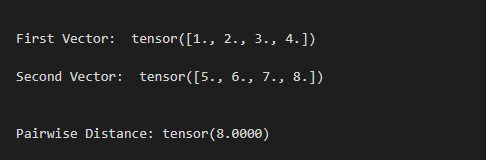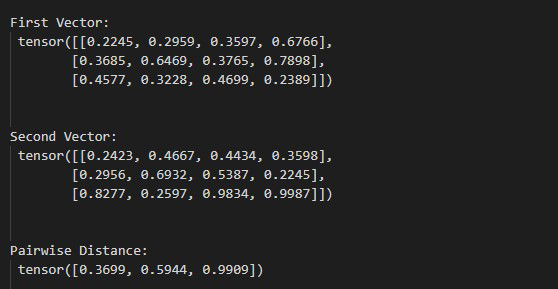Open In App

# How to Compute Pairwise Distance Between Two Vectors in PyTorch

In this article, we will discuss how to compute the pairwise distance between two vectors in PyTorch

The vector size should be the same and we can use PairwiseDistance() method to compute the pairwise distance between two vectors.

## PairwiseDistance() method

PairwiseDistance() method computes the pairwise distance between two vectors using the p-norm. This method is provided by the torch module. The below syntax is used to compute pairwise distance.

Syntax – torch.nn.PairwiseDistance(p=2)

Return – This method Returns the pairwise distance between two vectors.

Example 1:

The following program is to understand how to compute the pairwise distance between two vectors.

## Python3

 `import` `torch`` ` `# define first vector``vec1 ``=` `torch.tensor([``1.``, ``2.``, ``3.``, ``4.``])`` ` `# define second vector``vec2 ``=` `torch.tensor([``5.``, ``6.``, ``7.``, ``8.``])`` ` `# display tensors``print``(``"\n First Vector: "``, vec1)``print``(``"\n Second Vector: "``, vec2)`` ` `# define an instance of the PairwiseDistance``pdist ``=` `torch.nn.PairwiseDistance(p``=``2``)`` ` `# compute the pairwise distance``result ``=` `pdist(vec1, vec2)`` ` `# display result``print``(``"\n Pairwise Distance:"``, result)`

Output:Example 2:

## Python3

 `import` `torch`` ` `# define first vector``vec1 ``=` `torch.tensor([[``0.2245``, ``0.2959``, ``0.3597``, ``0.6766``],``                     ``[``0.3685``, ``0.6469``, ``0.3765``, ``0.7898``],``                     ``[``0.4577``, ``0.3228``, ``0.4699``, ``0.2389``]])`` ` `# define second vector``vec2 ``=` `torch.tensor([[``0.2423``, ``0.4667``, ``0.4434``, ``0.3598``],``                     ``[``0.2956``, ``0.6932``, ``0.5387``, ``0.2245``],``                     ``[``0.8277``, ``0.2597``, ``0.9834``, ``0.9987``]])`` ` `# display tensors``print``(``"\n First Vector: \n"``, vec1)``print``(``"\n Second Vector: \n"``, vec2)`` ` `# define an instance of the PairwiseDistance``pdist ``=` `torch.nn.PairwiseDistance(p``=``2``)`` ` `# compute the pairwise distance``result ``=` `pdist(vec1, vec2)`` ` `# display result``print``(``"\n Pairwise Distance: \n"``, result)`

Output: